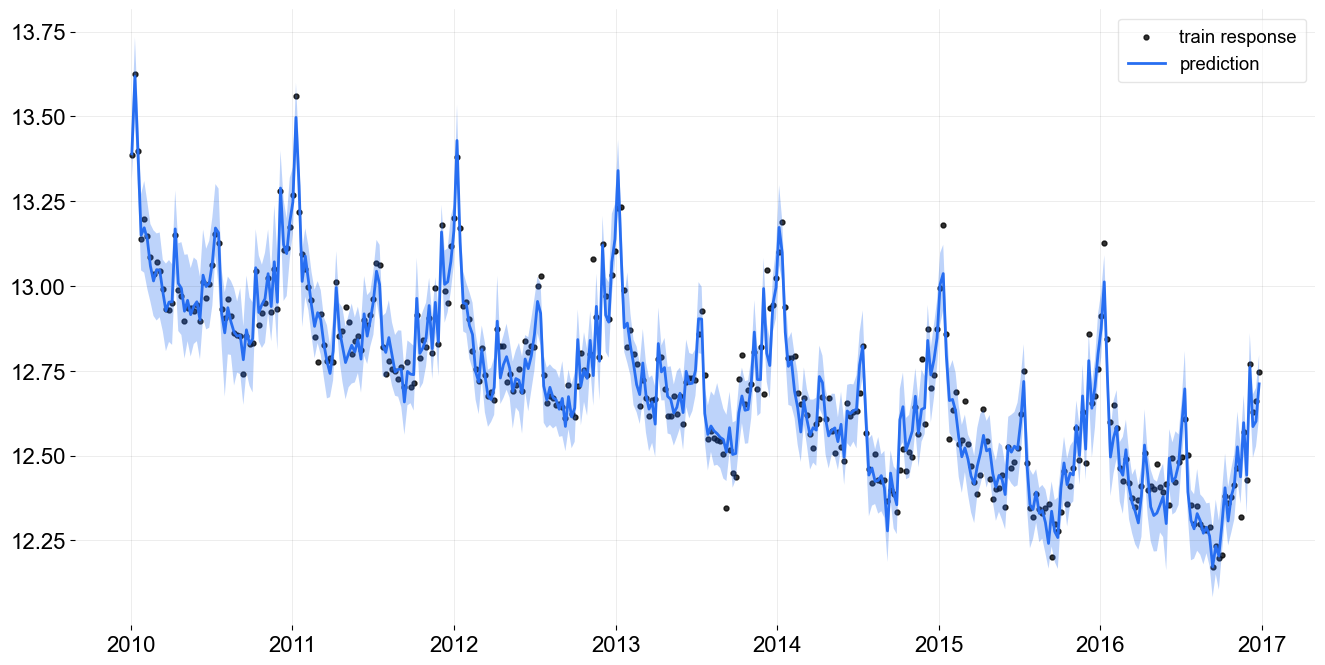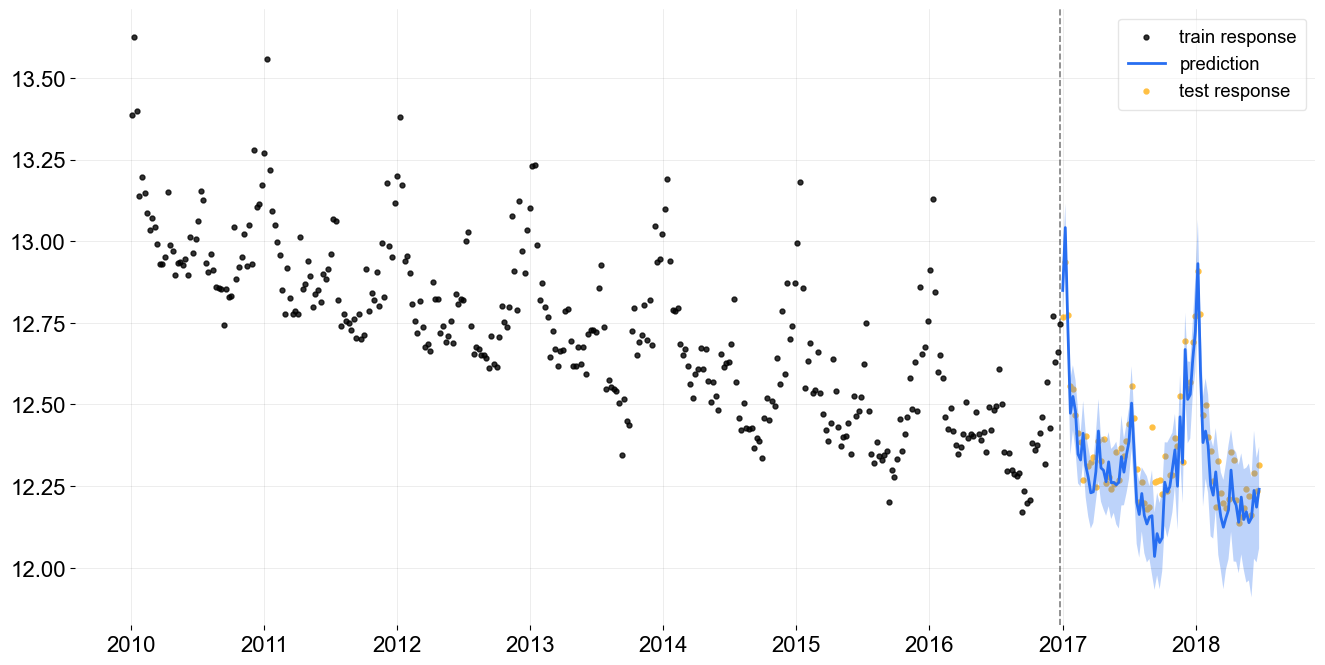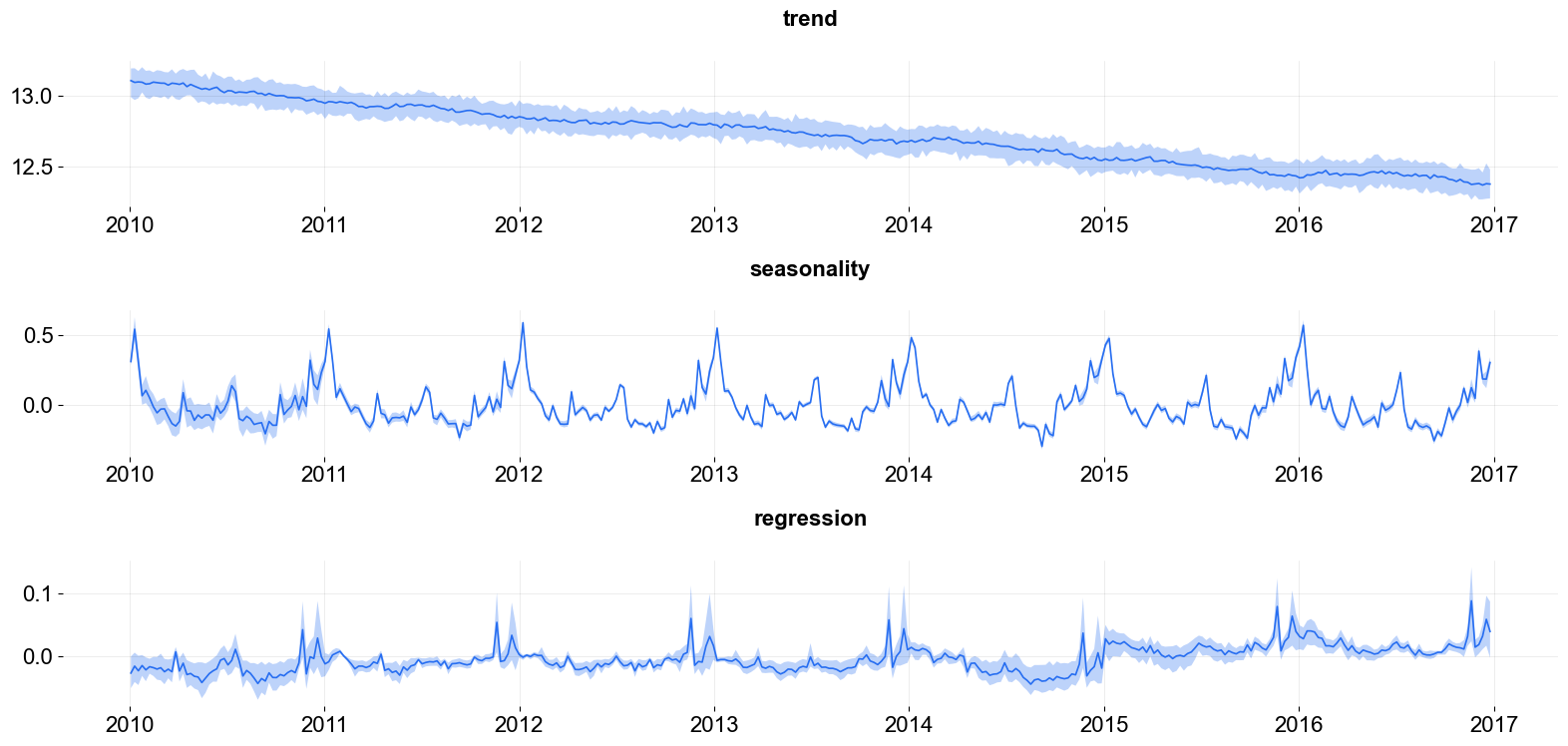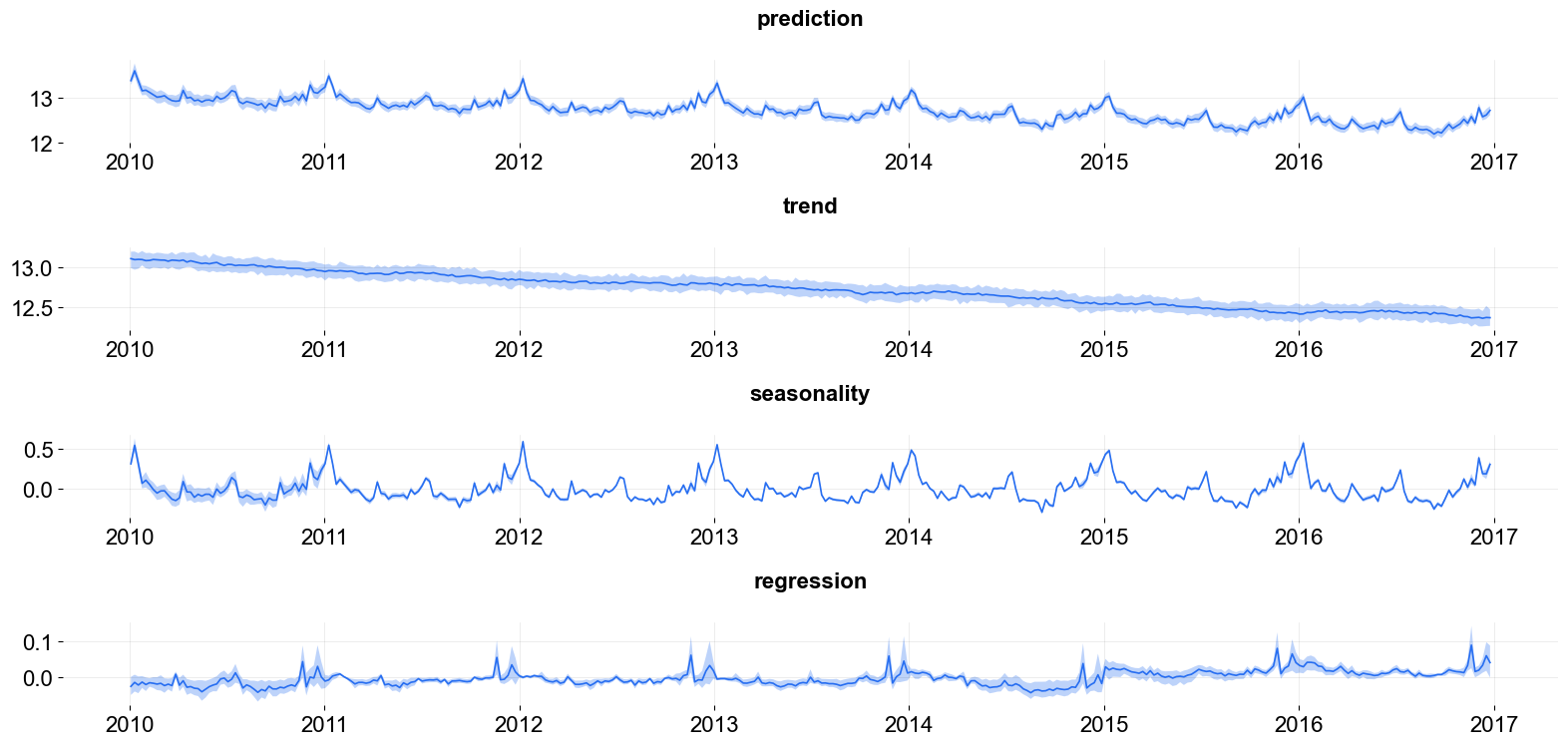# Prediction Decomposition¶

In this section, we will demonstrate how to visualize

• time series forecasting

• predicted components

by using the plotting utilities that come with the Orbit package.

:

%matplotlib inline

import pandas as pd
import numpy as np
import matplotlib.pyplot as plt

import orbit
from orbit.models import DLT
from orbit.diagnostics.plot import plot_predicted_data,plot_predicted_components

import warnings
warnings.filterwarnings('ignore')

:

print(orbit.__version__)

1.1.4

:

# load log-transformed data
train_df = df[df['week'] < '2017-01-01']
test_df = df[df['week'] >= '2017-01-01']

response_col = 'claims'
date_col = 'week'
regressor_col = ['trend.unemploy', 'trend.filling', 'trend.job']


## Fit a model¶

Here we use the DLTFull model as example.

:

dlt = DLT(
response_col=response_col,
regressor_col=regressor_col,
date_col=date_col,
seasonality=52,
prediction_percentiles=[5, 95],
)

dlt.fit(train_df)

2023-01-20 17:58:47 - orbit - INFO - Sampling (PyStan) with chains: 4, cores: 8, temperature: 1.000, warmups (per chain): 225 and samples(per chain): 25.



:

<orbit.forecaster.full_bayes.FullBayesianForecaster at 0x297725fa0>


## Plot Predictions¶

First, we do the prediction on the training data before the year 2017.

:

predicted_df = dlt.predict(df=train_df, decompose=True)

_ = plot_predicted_data(train_df, predicted_df,
date_col=dlt.date_col, actual_col=dlt.response_col)Next, we do the predictions on the test data after the year 2017. This plot is useful to help check the overall model performance on the out-of-sample period.

:

predicted_df = dlt.predict(df=test_df, decompose=True)

_ = plot_predicted_data(training_actual_df=train_df, predicted_df=predicted_df,
date_col=dlt.date_col, actual_col=dlt.response_col,
test_actual_df=test_df)## Plot Predicted Components¶

plot_predicted_components is the utility to plot each component separately. This is useful when one wants to look into the model prediction results and inspect each component separately.

:

predicted_df = dlt.predict(df=train_df, decompose=True)

_ = plot_predicted_components(predicted_df, date_col)One can use plot_components to have more components to be plotted if they are available in the supplied predicted_df.

:

_ = plot_predicted_components(predicted_df, date_col,
plot_components=['prediction', 'trend', 'seasonality', 'regression'])